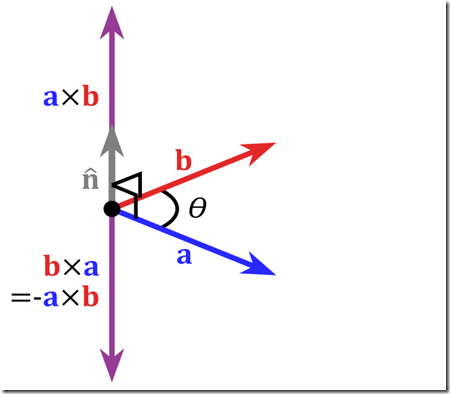# 三维向量的简单运算（点积、叉积及点到直线的距离）

V1( x1, y1, z1)·V2(x2, y2, z2) = x1*x2 + y1*y2 + z1*z2;

A·B = |A||B|Cos(θ)
θ是向量A和向量B见夹角。这里|A|我们称为向量A的模(norm)。这样我们就和容易计算两条线的夹角：

Cos(θ) = A·B /(|A|*|B|)

n = u x v;i,j,k.

i,j,k满足以下特点

i = j x k; j = k x i; k = i x j;

k x j = –i; i x k = –j; j x i = –k;

i x i = j x j = k x k = 0; (0是指0向量）

u = Xu*i + Yu*j + Zu*k;

v = Xv*i + Yv*j + Zv*k;

= Xu*Xv*(ixi) + Xu*Yv*(i x j) + Xu*Zv*(i x k) + Yu*Xv*(j x i) + Yu*Yv*(j x j) + Yu*Zv*(j x k) + Zu*Xv*( k x i ) + Zu*Yv(k x j) + Zu*Zv(k x k)

u x v = (Yu*Zv – Zu*Yv)*i + (Zu*Xv – Xu*Zv)j + (Xu*Yv – Yu*Xv)k;

叉乘表示垂直于uxv的右向量。

可以通过叉乘，修正向量关系，从而构建坐标系。 常见的有 摄相机矩阵和TBN空间转换矩阵的构建。

d = (AB x AC)/|AB|

(AB X AC)/2是三角形ABC的面积，这个三角形的底是|AB|，高就是CAB的距离。有时叉积得到的是一个负值，这种情况下距离就是上述结果的绝对值。

设某三维平面表达式为
a*x+b*y+c*z+d = 0;

(a*x1 + b*y1 + c*z1+d) / (a*a + b*b +c*c)